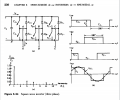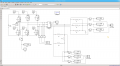# Tri-Phase Full-Bridge Thyristor based rectifier

#### PsySc0rpi0n

Joined Mar 4, 2014
1,446
Hello...

I'm analysing a Tri-Phase Full-Bridge Thyristor based rectifier in Matlab Simulink. I have searched for the circuit schematic and assembled it.

Now I'm being asked to plot a couple of values based on an image from a book we are following in classes that is Ned Mohan's book.

Teacher is asking us to plot:

$$v_{An}$$
$$i_{A}$$
$$i_{DA+}$$
$$i_{D}$$

following picture 8.24 from Ned Mohan's books which is the followingThe circuit I'm simulating in Matlab Simulink is the following:My question is if the spot in the circuit where I have Scope V_An is the correct spot for the asked VAn voltage? And for 'id' current? Would it be the current out of Vdc?